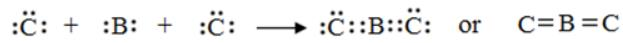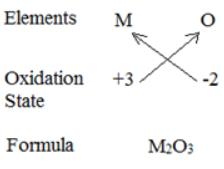Courses

# Test: Periodic Classification of Elements (Hard)

## 20 Questions MCQ Test | Test: Periodic Classification of Elements (Hard)

Description
This mock test of Test: Periodic Classification of Elements (Hard) for Class 10 helps you for every Class 10 entrance exam. This contains 20 Multiple Choice Questions for Class 10 Test: Periodic Classification of Elements (Hard) (mcq) to study with solutions a complete question bank. The solved questions answers in this Test: Periodic Classification of Elements (Hard) quiz give you a good mix of easy questions and tough questions. Class 10 students definitely take this Test: Periodic Classification of Elements (Hard) exercise for a better result in the exam. You can find other Test: Periodic Classification of Elements (Hard) extra questions, long questions & short questions for Class 10 on EduRev as well by searching above.
QUESTION: 1

### The elements A, B, and C belong to group 2, 14, and 16 respectively, of the periodic table. Which of the two elements will form covalent bonds?

Solution: The covalent bond is formed by the sharing of electrons between two atoms. As the element B (which belongs to group 14) has 4 valence electrons which it can share with two elements of C type (from group 16) electrons to complete the octet of each included atomQUESTION: 2

### Which of the following does not decrease while moving down the group of the periodic table?

Solution: Number of valence electrons in a group remain the same.
QUESTION: 3

### An element X belongs to the 3rd period and 1st group of the periodic table. What is the number of valence electrons in its atom?

Solution: As the element belongs to the 1st group of the periodic table, so the number of valence electrons in its atom is one.
QUESTION: 4

An element M is in group 13th of the periodic table, the formula for its oxide is

Solution: As the element M belongs to group 13th of the periodic table so it has 3 valence electrons, i.e., it can have +3 oxidation state while oxygen atom (with 2 valency) has −2 oxidation state. So the formula for the corresponding oxide is M2O3.QUESTION: 5

Consider The Following Statements And Choose The Incorrect One:

Solution: Here, all the statements are incorrect. Hence, your answer will be option (d). In option (a), In Mendeleev’s periodic table, the elements are arranged on the basis of their atomic masses and also on the similarity of chemical properties, whereas the modern periodic table is based on the atomic numbers of elements. In option (b): Dobereiner’s triads also exist in the columns of Newlands’ classification of elements based on the law of octaves. In option (c): The periodic table is a chart of elements prepared in such a way that the elements having similar properties occur in the same vertical column or group. It is called periodic because the elements having similar properties are repeated after certain intervals or periods, and it is called a table because the elements are arranged in the tabular form.
QUESTION: 6

Arrange The Following Elements In The Order Of Their Increasing Nonmetallic Character: Li, O, C, Be, F

Solution: Li, O, C, Be, F belong to same period i.e. 2nd period. In a period on moving from left to right, non-metallic character increases. Thus, the order of increasing non-metallic character is: Li < Be < C < O < F.
QUESTION: 7

Which Of The Following Are The Characteristics Of Isotopes Of An Element?

Solution: Isotopes are the atoms of the same element having same atomic number and hence, similar chemical properties but different atomic masses. Note: All the isotopes of an element have the same atomic number. Therefore, they can be placed at one place in the same group of the periodic table. Chemical properties depend on the electronic configuration of the element i.e. atomic number of the element.
QUESTION: 8

Which One Of The Following Does Not Increase While Moving Down The Group Of The Periodic Table?

Solution: All the elements of a group of the periodic table have the same number of valence electrons. e.g., all the elements of group 1 have one valence electron each in their atoms. All the elements of group 18 have 8 valence electrons each in their atoms, except helium which has only 2 valence electrons in its atom. All the remaining three properties increase down the group. Note: On moving down in a particular group of the periodic table, the number of valence electrons in the elements remains the same. All the remaining three properties increase down the group.
QUESTION: 9

Which Of The Following Is The Outermost Shell For Elements Of Period 2?

Solution: The elements of 2nd period involve the filling of 2nd shell i.e. L shell. Because in periods 2, there are two shells, K and L.
QUESTION: 10

Where Would You Locate The Element With Electronic Configuration 2, 8 In The Modern Periodic Table?

Solution: Element with electronic configuration 2, 8, is an inert gas i.e., neon and hence, belongs to group 18.

Note: Group 18 elements are known as noble gases or inert gases. They are helium, neon, argon, krypton, etc. All the noble gases have 8 electrons in their outermost shell (except helium which has only 2 electrons) in the K valence shell. Thus, the valence shells of all the noble gases are completely filled with electrons.

QUESTION: 11

The Elements A, B, C, D, and E Have Atomic Number 9, 11, 17, 12, and 13 Respectively. Which Pair Of Elements Belongs To The Same Group?

Solution: Electronic configuration of A (atomic number 9) is 2, 7. Electronic configuration of B (atomic number 11) is 2, 8, 1. Electronic configuration of C (atomic number 17) is 2, 8, 7. Electronic configuration of D (atomic number 12) is 2, 8, 2. Electronic configuration of E (atomic number 13) is 2, 8, 3. Elements which differ in atomic number by 8 i.e., 9 (A fluorine) and 17 (Cl chlorine) lie in the same group i.e., group 17 (halogens).
QUESTION: 12

In A Science Quiz Competition, Simran Are Asked A Question Where She Had To Choose The Statement Which Was/Were Incorrect?

Solution: The groups 1 and 2, and 13 to 17 contain the normal elements (typical elements or representative elements). Group 18 elements are known as noble gases or inert gases. They are helium, neon, argon, krypton, etc. All the noble gases have 8 electrons in their outermost shells (except helium which has only 2 electrons in the K valence shell). Thus, the valence shells of all the noble gases are completely filled with electrons.
QUESTION: 13

According To Mendeleev’s Periodic Law, The Elements Were Arranged In The Periodic Table In The Order Of

Solution: According to Mendeleev’s periodic law, the elements were arranged in the periodic table in the order of increasing atomic masses.

Note: Mendeleev’s periodic law states that the physical and chemical properties of elements are a periodic function of their atomic masses.

QUESTION: 14

Upto Which Element, The Law Of Octaves Was Found To Be Applicable.

Solution: Newlands’ law of octaves was applicable only to lighter elements having atomic masses upto 40 u i.e., upto calcium. After calcium, every eighth element did not possess properties similar to that of the first element.
QUESTION: 15

Read The Following Sentences Carefully, And Choose The Incorrect One(S):

Solution: The first period starts with hydrogen and ends with noble gas helium. All other periods start with alkali metals like lithium, sodium, potassium, etc., and end with noble gases like neon, argon, krypton, etc.
QUESTION: 16

Which Of The Following Gives The Correct Increasing Order Of The Atomic Radii Of O, F, and N?

Solution: As we move from left to right in a period, the atomic number of each succeeding element increases by 1. So, the electrons are attracted close to the nucleus, and hence, the atomic size decreases, along a period from left to right. Therefore, the increasing order of radii is F, O, N as atomic number of F, O, and N are 9, 8, and 7 respectively. Due to which F belongs to 17th group. O belongs to 16th group and N belongs to 15th group. Note: Atomic radii is the distance between the centre of the nucleus and the outermost shell which contains electrons in an isolated system.
QUESTION: 17

The law of octaves is given by ___.

Solution: Law of octaves, in chemistry, the generalization made by the English chemist J.A.R. Newlands in 1865 that, if the chemical elements are arranged according to increasing atomic weight, those with similar physical and chemical properties occur after each interval of seven elements.
QUESTION: 18

Which of the following elements will form an acidic oxide?

Solution: Element with atomic number 7 has electron configuration 2,5 which means it can gain 3 electrons and is an electronegative element. It should be a non-metal and non-metals will form acidic oxide.
QUESTION: 19

The element with atomic number 14 is hard and forms acidic oxide and a covalent halide. To which of the following categories does the element belong?

Solution: Element with atomic no. 14 is Silicon and is a metalloid. It forms an acidic oxide, thus behaving as a non-metal, and also forms covalent halide, thus acting as a metal.
QUESTION: 20

Which of the following set of elements is written in order of their increasing metallic character?

Solution: Metallic character increase as we move down the group. In the elements that belong to the same group Berrylium is at the top and Calcium is at the bottom.